SYLLABUS  Previous: 1.4.1 Convergence, Richardson extrapolation  Up: 1.4 Numerical discretization  Next: 1.4.3 Finite elements

### 1.4.2 Sampling on a regular grid

The main advantage of sampling data on a grid is its simplicity: starting from a continuous function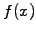a finite number of values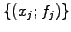are measured on what is generally a homogeneous mesh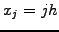,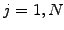. The values at the domain boundary appear explicitly as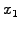and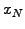.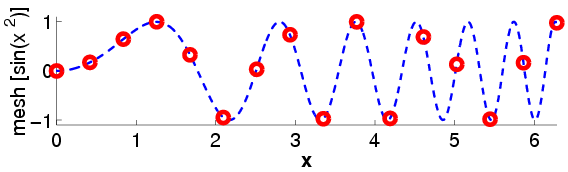Figure (1.4.2#fig.1) shows that the sampled function is unknown almost everywhere, except on the grid points where it is exact. If the sampling is dense enough and the function smooth, intermediate values can be interpolated from neighboring data using a Taylor expansion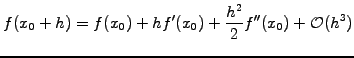(1.4.2#eq.1)

Combining Taylor expansions from the same function evaluated on neighboring locations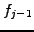,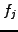,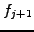,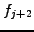, etc. For example, the difference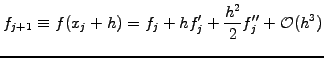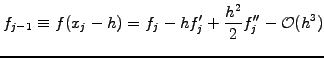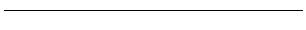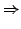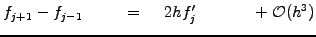leads to the finite difference approximations that has previously been used in sect.1.2.1. Along the same lines, you can derive the formula for the k-th derivative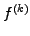from Abramowitz  §25.1.2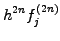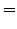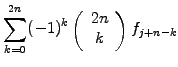(1.4.2#eq.2)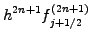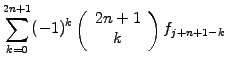(1.4.2#eq.3)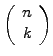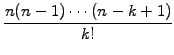Remember that a discretization on a grid is non-compact: the convergence depends not only on the initial discretization, but also on the interpolation that is required afterwards to reconstruct the data between the mesh points.

SYLLABUS  Previous: 1.4.1 Convergence, Richardson extrapolation  Up: 1.4 Numerical discretization  Next: 1.4.3 Finite elements